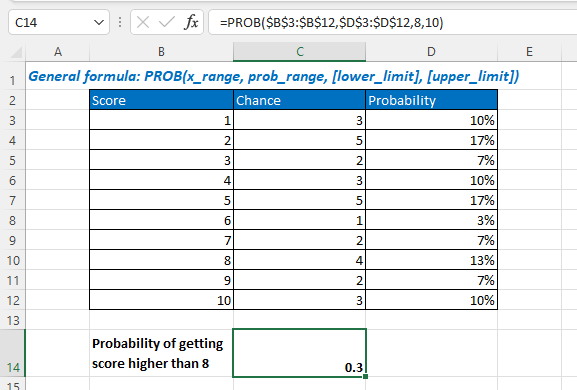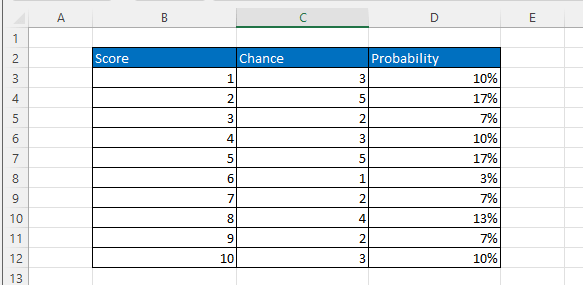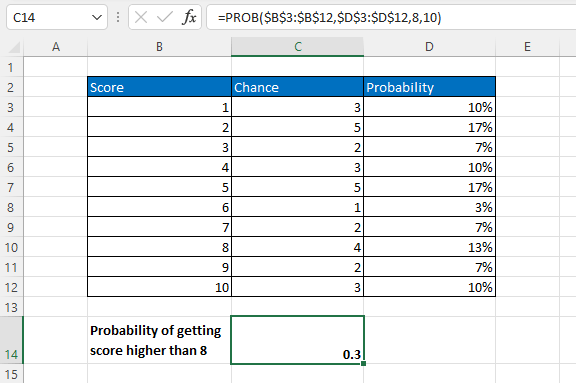## Excel PROB Function### Description

The Excel PROB function returns the probability that values in a range are between two limits, which is frequently used to estimate business losses or used by a risk manager to analyze the financial probability.

Formula syntax

PROB(x_range,prob_range,[lower_limit],[upper_limit])

Arguments

 x_range: Required, the range of numeric values of x with which there are associated probabilities. prob_range: Required, the set or array of probabilities that are associated with values in x_range. The array must be in the same length as x_range array, and the values in prob_range must add up to 1. lower_limit: Optional, the lower boundary of the value for which you want a probability. upper_limit: Optional, the upper boundary of the value for which you want a probability.

### Returns

PROB function returns a numeric value.

### Remarks

1. Errors appear when

• Any value in prob_range ≤ 0 or any value in prob_range > 1, PROB function returns the #NUM error value.
• The sum of values in prob_range is not equal to 1, PROB function returns the #NUM error value.
• X_range and prob_range have different numbers of data points, PROB function return the #N/A error value.

2. if upper_limit is omitted, PROB function returns the probability that values in x_range are equal to lower_limit.

### Version

Excel 2007 and later versions

### Usage and Examples

Basic usage

Supposing a table that contains the scores, the chances of getting each score, and the probabilities of getting each score as below screenshot shown:To get the probability of getting a score higher than 8 based on the table, please use the PROB function as below:

=PROB(\$B\$3:\$B\$12,\$D\$3:\$D\$12,8,10)

Press Enter key to get the probability.#### Other Functions:

• Excel POISSON Function
The Excel POISSON function returns the Poisson distribution which is used to predict the number of events occurring over a specific time.

• Excel FDIST Function
The Excel FDIST Function returns the (right-tailed) F probability distribution which is usually used to measure the degree of diversity between two data sets.

• Excel EXPON.DIST Function
EXPON.DIST function used in Excel 2010, returns the exponential distribution based on the given x value and the parameter of the distribution.

• Excel F.DIST Function
The Excel F.DIST function returns the F probability distribution which is usually used to measure the degree of diversity between two data sets.

### The Best Office Productivity Tools

#### Kutools for Excel - Helps You To Stand Out From Crowd

 Popular Features: Find, Highlight or Identify Duplicates  |  Delete Blank Rows  |  Combine Columns or Cells without Losing Data  |  Round without Formula ... Super VLookup: Multiple Criteria  |  Multiple Value  |  Across Multi-Sheets  |  Fuzzy Lookup... Adv. Drop-down List: Easy Drop Down List  |  Dependent Drop Down List  |  Multi-select Drop Down List... Column Manager: Add a Specific Number of Columns  |  Move Columns  |  Toggle Visibility Status of Hidden Columns  |  Compare Columns to Select Same & Different Cells ... Featured Features: Grid Focus  |  Design View  |  Big Formula Bar  |  Workbook & Sheet Manager | Resource Library (Auto Text)  |  Date Picker  |  Combine Worksheets  |  Encrypt/Decrypt Cells  |  Send Emails by List  |  Super Filter  |  Special Filter (filter bold/italic/strikethrough...) ... Top 15 Toolsets:  12 Text Tools (Add Text, Remove Characters ...)  |  50+ Chart Types (Gantt Chart ...)  |  40+ Practical Formulas (Calculate age based on birthday ...)  |  19 Insertion Tools (Insert QR Code, Insert Picture from Path ...)  |  12 Conversion Tools (Numbers to Words, Currency Conversion ...)  |  7 Merge & Split Tools (Advanced Combine Rows, Split Excel Cells ...)  |  ... and more

Kutools for Excel Boasts Over 300 Features, Ensuring That What You Need is Just A Click Away...#### Office Tab - Enable Tabbed Reading and Editing in Microsoft Office (include Excel)

• One second to switch between dozens of open documents!
• Reduce hundreds of mouse clicks for you every day, say goodbye to mouse hand.
• Increases your productivity by 50% when viewing and editing multiple documents.
• Brings Efficient Tabs to Office (include Excel), Just Like Chrome, Edge and Firefox.